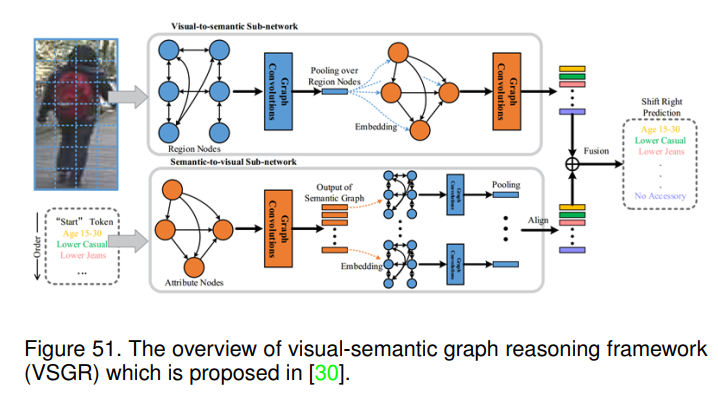# 行人属性识别研究综述（二）

### 文章目录

• 6 PAR（行人属性识别）算法综述
• 6.1全局基于图像的模型
• 6.1.1 ACN (iccvw-2015)
• 6.1.2 DeepSAR and DeepMAR (ACPR-2015) 
• 6.1.3 MTCNN (TMM-2015) 
• 6.2 基于部件的模型
• 6.2.1 Poselets (ICCV-2011)
• 6.2.3 PANDA (cvp -2014) 
• 6.2.4 MLCNN (ICB-2015) 
• 6.2.5 AAWP (ICCV-2015) 
• 6.2.6 ARAP (BMVC2016) 
• 6.2.7 DeepCAMP (CVPR-2016) 
• 6.2.8 PGDM (ICME-2018) 
• 6.2.9 DHC (eccv-2016) 
• 6.2.10 LGNet (BMVC-2018) 
• 6.3 基于注意力的模型
• 6.3.1 HydraPlus-Net (ICCV-2017)
• 6.3.2 VeSPA (ArXiv-2017) 
• 6.3.3 DIAA (ECCV-2018) 
• 6.3.4 CAM (PRL-2017) 
• 6.4 基于序列预测的模型
• 6.4.1 cnn-rnn (cvpr-2016)
• 6.4.2 JRL (ICCV-2017) 
• 6.4.3 GRL (IJCAI-2018) 
• 6.4.4 JCM (arXiv-2018) 
• 6.4.5 RCRA (AAAI-2019) 
• 6.5 基于损失函数的模型
• 6.5.1 WPAL-network (BMVC-2017)
• 6.5.2 AWMT (MM-2017) 
• 6.6 基于算法的课程学习
• 6.6.1 MTCT (wacv-2017) 
• 6.6.2 CILICIA (ICCV-2017) 
• 6.7 基于图模型的算法
• 6.7.1 DCSA (ECCV-2012) 
• 6.7.2 A-AOG (TPAMI-2018) 
• 6.7.3 VSGR (AAAI-2019) 
• 6.8 其他算法
• 6.8.1 PatchIt (BMVC-2016) 
• 6.8.2 FaFS (CVPR-2017)
• 6.8.3 GAM (AVSS-2017) 
• 7 应用
• 8 未来研究方向
• 8.1更精确高效的局部定位算法
• 8.2用于数据增强的深度生成模型
• 8.3进一步探索视觉注意机制
• 8.4 新设计的损失函数
• 8.5 探索更高级的网络架构
• 8.6 先验知识引导学习
• 8.7 多模态行人属性识别
• 8.8 基于视频的行人属性识别
• 8.9 属性与其他任务的联合学习
• 9 结论

🐇🐇🐇🐇🐇🐇
🐇 欢迎阅读 【AI浩】 的博客🐇
👍 阅读完毕，可以动动小手赞一下👍
🌻 发现错误，直接评论区中指正吧🌻
📆 这是一篇研究行人属性识别综述论文📆
💯论文链接：Pedestrian Attribute Recognition: A Survey💯

## 6 PAR（行人属性识别）算法综述## 6.1全局基于图像的模型

### 6.1.1 ACN (iccvw-2015)KL(P∥Q)=∑iNP(xi)log⁡Q(xi)P(xi)(11)K L(P \| Q)=\sum_{i}^{N} P\left(x_{i}\right) \log \frac{Q\left(x_{i}\right)}{P\left(x_{i}\right)} \tag{11}

## 6.1.2 DeepSAR and DeepMAR (ACPR-2015)Lce=−1N∑i=1N∑l=1Lyillog⁡(P^il)+(1−yil)log⁡(1−pil^)(12)L_{c e}=-\frac{1}{N} \sum_{i=1}^{N} \sum_{l=1}^{L} y_{i l} \log \left(\hat{P}_{i l}\right)+\left(1-y_{i l}\right) \log \left(1-\hat{p_{i l}}\right) \tag{12}

pil^=11+exp⁡(−xil)(13)\hat{p_{i l}}=\frac{1}{1+\exp \left(-x_{i l}\right)}\tag{13}

Lwce=−1N∑i=1N∑l=1Lwl(yillog⁡(Pil^)+(1−yil)log⁡(1−pil^))(14)L_{w c e}=-\frac{1}{N} \sum_{i=1}^{N} \sum_{l=1}^{L} w_{l}\left(y_{i l} \log \left(\hat{P_{i l}}\right)+\left(1-y_{i l}\right) \log \left(1-\hat{p_{i l}}\right)\right) \tag{14}

wl=exp⁡(−pl/σ2)(15)w_{l}=\exp \left(-p_{l} / \sigma^{2}\right) \tag{15}

### 6.1.3 MTCNN (TMM-2015)min⁡L,S∑m=1M∑i=1Nm12[max⁡(0,1−Ymi(Lsm)TXmi)]2+μ∑k=1K∑g=1G∥skg∥2+γ∥L∥1+λ∥L∥F2(16)\begin{array}{r} \min _{L, S} \sum_{m=1}^{M} \sum_{i=1}^{N_{m}} \frac{1}{2}\left[\max \left(0,1-Y_{m}^{i}\left(L s^{m}\right)^{T} X_{m}^{i}\right)\right]^{2}+ \\ \mu \sum_{k=1}^{K} \sum_{g=1}^{G}\left\|s_{k}^{g}\right\|_{2}+\gamma\|L\|_{1}+\lambda\|L\|_{F}^{2} \end{array} \tag{16}

## 6.2 基于部件的模型

### 6.2.1 Poselets (ICCV-2011)### 6.2.3 PANDA (cvp -2014) 

Zhang等人发现与某些属性相关的信号是细微的，图像受姿态和视角的影响。对于佩戴眼镜的属性，在整个人的尺度上信号较弱，且外观随着头部姿态、框架设计和头发遮挡的变化而显著变化。他们认为准确预测基础属性的关键在于定位对象部件并建立它们与模型部件的对应关系。他们提出联合使用全局图像块和局部块进行行人属性识别，整体流程如图27 (a)和(b)所示。### 6.2.4 MLCNN (ICB-2015)### 6.2.5 AAWP (ICCV-2015)### 6.2.6 ARAP (BMVC2016)### 6.2.7 DeepCAMP (CVPR-2016)### 6.2.8 PGDM (ICME-2018) 

PGDM是第一个尝试探索行人身体结构知识(即行人姿态)进行行人属性学习的工作。他们首先使用预训练的姿态估计模型估计给定人体图像的关键点。然后根据这些关键点提取零件区域;分别提取局部区域和整幅图像的深度特征，独立用于属性识别。然后将这两个分数融合在一起，实现最终的属性识别。姿态估计的可视化和PGDM的整个流程分别如图32 (a)和(b)所示。### 6.2.9 DHC (eccv-2016)### 6.2.10 LGNet (BMVC-2018)## 6.3 基于注意力的模型

### 6.3.1 HydraPlus-Net (ICCV-2017)HP-net以分阶段的方式进行训练，即M-net、AF-net以及剩余的GAP和fc层以顺序的方式进行训练。输出层用于最小化交叉熵损失和softmax损失，分别进行行人属性识别和行人重识别。

### 6.3.2 VeSPA (ArXiv-2017)VeSPA将视图信息考虑在内，以更好地估计相应的属性。这是因为作者发现暗示属性的视觉线索具有很强的局部性，可以对人的属性(如头发、背包、短裤等)进行推断。高度依赖于行人获得的视角。如图36所示，将图像输入到inception (K层)并获得其特征表示。引入视图相关单元将特征映射映射为粗属性预测y^att=[y1,y2,…,yc]T\hat{y}_{a t t}=\left[y^{1}, y^{2}, \ldots, y^{c}\right]^{T}。然后，使用视图预测器估计视图权重y^view。注意力权重用于相乘特定视图的预测，并获得最终的多类属性预测Y^c=[y1,y2,…,yC]T\hat{Y}_{c}=\left[y^{1}, y^{2}, \ldots, y^{C}\right]^{T}

### 6.3.3 DIAA (ECCV-2018) 

DIAA算法可以看作是一种用于行人属性识别的集成方法。如图37所示，他们的模型包含以下模块:用于深度不平衡分类的多尺度视觉注意和加权焦点损失。在多尺度视觉注意方面，从图37中可以看出，作者采用了不同层的特征图。他们提出了加权焦点损失函数来衡量预测属性向量和真实值之间的差异:
Lw(y^p,y)=−∑c=1Cwc((1−σ(y^pc))γlog⁡(σ(y^pc))yc+σ(y^pc)γlog⁡(1−σ(y^pc))(1−yc)),(17)\begin{array}{r} \mathcal{L}_{w}\left(\hat{y}_{p}, y\right)=-\sum_{c=1}^{C} w_{c}\left(\left(1-\sigma\left(\hat{y}_{p}^{c}\right)\right)^{\gamma} \log \left(\sigma\left(\hat{y}_{p}^{c}\right)\right) y^{c}+\right. \\ \left.\sigma\left(\hat{y}_{p}^{c}\right)^{\gamma} \log \left(1-\sigma\left(\hat{y}_{p}^{c}\right)\right)\left(1-y^{c}\right)\right), \end{array} \tag{17}Lai(y^ai,y)=(1+std⁡s(H))Lb(y^ai,y)\mathcal{L}_{a_{i}}\left(\hat{y}_{a_{i}}, y\right)=\left(1+\operatorname{std}_{s}(H)\right) \mathcal{L}_{b}\left(\hat{y}_{a_{i}}, y\right)

### 6.3.4 CAM (PRL-2017)L=1Neα(PijM+βμ)L=\frac{1}{N} e^{\alpha\left(P_{i j}^{M}+\beta \mu\right)}

## 6.4 基于序列预测的模型

### 6.4.1 cnn-rnn (cvpr-2016)s(t)=UlTxts(t)=U_{l}^{T} x_{t}

l1,…,lk=arg⁡max⁡l1,…,lkP(l1,…,lk∣I)=arg⁡max⁡l1,…,lkP(l1∣I)×P(l2∣I,l1)…P(lk∣I,l1,…,lk−1)(21)\begin{array}{r} l_{1}, \ldots, l_{k}=\arg \max _{l_{1}, \ldots, l_{k}} P\left(l_{1}, \ldots, l_{k} \mid I\right) \\ =\arg \max _{l_{1}, \ldots, l_{k}} P\left(l_{1} \mid I\right) \times P\left(l_{2} \mid I, l_{1}\right) \ldots P\left(l_{k} \mid I, l_{1}, \ldots, l_{k-1}\right) \end{array} \tag{21}

CNN-RNN模型与图像描述任务中使用的深度模型非常相似。它们都将一张图像作为输入，并在编码器-解码器框架下输出一系列单词。主要的区别在于，caption模型输出一句话，而CNN-RNN模型生成属性(但这些属性也相互关联)。因此，可以借鉴图像描述社区的一些技术来帮助提高行人属性识别的性能。

### 6.4.2 JRL (ICCV-2017)### 6.4.3 GRL (IJCAI-2018) 

GRL是在JRL的基础上发展起来的，它也采用RNN模型对人的属性进行顺序预测。与JRL不同的是，GRL是以群体为单位逐步识别人的属性，同时关注群体内和群体间的关系。如图41(1)所示，由于组内属性互斥且组间存在关系，作者将整个属性列表划分为多个组。例如，BoldHair和BlackHair不能出现在同一个人图像上，但它们都与一个人的头肩区域有关，可以在同一组中一起被识别。它是一种不需要预处理的端到端单模型算法，同时利用了分组行人属性之间更多潜在的组内和组间依赖关系。整体算法如图41(2)所示。### 6.4.4 JCM (arXiv-2018)### 6.4.5 RCRA (AAAI-2019)Ft=sigmoid⁡(Ht)⊗F+F(22)F_{t}=\operatorname{sigmoid}\left(H_{t}\right) \otimes F+F \tag{22}

## 6.5 基于损失函数的模型

### 6.5.1 WPAL-network (BMVC-2017)Loss⁡wce=∑i=1L12wi∗pi∗log⁡(p^i)+12(1−wi)(1−pi)∗log⁡(1−p^i)(23)\operatorname{Loss}_{w c e}=\sum_{i=1}^{L} \frac{1}{2 w_{i}} * p_{i} * \log \left(\hat{p}_{i}\right)+\frac{1}{2\left(1-w_{i}\right)}\left(1-p_{i}\right) * \log \left(1-\hat{p}_{i}\right) \tag{23}

### 6.5.2 AWMT (MM-2017)Θ=arg⁡min⁡Θ∑j=1M∑i=1N<λj,L(ψj(Ii;Θ)−Lij)>(24)\Theta=\arg \min _{\Theta} \sum_{j=1}^{M} \sum_{i=1}^{N}<\lambda_{j}, \mathcal{L}\left(\psi_{j}\left(\mathbf{I}_{i} ; \Theta\right)-\mathbf{L}_{i j}\right)> \tag{24}

## 6.6 基于算法的课程学习

### 6.6.1 MTCT (wacv-2017)Lt−STE=∑It,Ips,Ins∈Tlog⁡(1+∥ft(It)−fs(Ips)∥2α)β(1+∥ft(It)−fs(Ips)∥2α)β+(1+∥ft(It)−fs(Ins)∥2α)β(25)\begin{array}{r} L_{t-S T E}=\sum_{I_{t}, I_{p s}, I_{n s} \in T} \\ \log \frac{\left(1+\frac{\left\|f_{t}\left(I_{t}\right)-f_{s}\left(I_{p s}\right)\right\|^{2}}{\alpha}\right)^{\beta}}{\left(1+\frac{\left\|f_{t}\left(I_{t}\right)-f_{s}\left(I_{p s}\right)\right\|^{2}}{\alpha}\right)^{\beta}+\left(1+\frac{\left\|f_{t}\left(I_{t}\right)-f_{s}\left(I_{n s}\right)\right\|^{2}}{\alpha}\right)^{\beta}} \end{array} \tag{25}

### 6.6.2 CILICIA (ICCV-2017)pi=∑j=1,j≠iTcov⁡(yti,ytj)σ(ytiσ(ytj)),i=1,…,T(26)p_{i}=\sum_{j=1, j \neq i}^{T} \frac{\operatorname{cov}\left(y_{t_{i}}, y_{t_{j}}\right)}{\sigma\left(y_{t_{i}} \sigma\left(y_{t_{j}}\right)\right)}, i=1, \ldots, T \tag{26}

Lt=1N∑i=1N∑j=1M(1/Mj∑n=1M1/Mn)⋅1[yi=j]⋅log⁡(pi,j)(27)L_{t}=\frac{1}{N} \sum_{i=1}^{N} \sum_{j=1}^{M}\left(\frac{1 / M_{j}}{\sum_{n=1}^{M} 1 / M_{n}}\right) \cdot \mathbb{1}\left[y_{i}=j\right] \cdot \log \left(p_{i, j}\right) \tag{27}

Lw=λ⋅Ls+(1−λ)⋅Lwf(28)L_{w}=\lambda \cdot L_{s}+(1-\lambda) \cdot L_{w}^{f} \tag{28}

## 6.7 基于图模型的算法

### 6.7.1 DCSA (ECCV-2012)### 6.7.2 A-AOG (TPAMI-2018) 

A-AOG模型(attribute and - or grammar)显式地表示了人体各部位的分解和连接，并考虑了姿态和属性之间的关联。该算法基于and- or图，and-节点表示分解或依赖;or节点表示分解或部件类型的可选选择。具体来说，它主要整合了三种语法:短语结构语法、依存语法和一个属性语法。A−AOG=⟨S,V,E,X,P⟩(29)A-A O G=\langle S, V, E, X, \mathcal{P}\rangle \tag{29}

pg=(V(pg),E(pg),X(pg))(30)p g=(V(p g), E(p g), X(p g)) \tag{30}P(pg∣I;λ)∝P(I∣pg;λ)P(pg;λ)=1Zexp⁡{−E(I∣pg;λ)−E(pg;λ)}(31)\begin{aligned} & P(p g \mid I ; \lambda) \propto P(I \mid p g ; \lambda) P(p g ; \lambda) \\ = & \frac{1}{Z} \exp \{-\mathcal{E}(I \mid p g ; \lambda)-\mathcal{E}(p g ; \lambda)\} \end{aligned} \tag{31}

P(pg∣I;λ)=1Zexp⁡{−EappV(I∣pg;λ)−EappX(I∣pg;λ)−Erel V(pg;λ)−Erel X(pg;λ)}(32)\begin{array}{r} P(p g \mid I ; \lambda)=\frac{1}{Z} \exp \left\{-\mathcal{E}_{a p p}^{V}(I \mid p g ; \lambda)-\mathcal{E}_{a p p}^{X}(I \mid p g ; \lambda)\right. \\ \left.-\mathcal{E}_{\text {rel }}^{V}(p g ; \lambda)-\mathcal{E}_{\text {rel }}^{X}(p g ; \lambda)\right\} \end{array} \tag{32}

S(pg∣I)=−EappV(I∣pg)−Eapp X(I∣pg)−Erel V(pg)−Erel X(pg)=SappV(I,pg)+SappX(I,pg)+Srel V(pg)+Srel X(pg)(33)\begin{aligned} S(p g \mid I) & =-\mathcal{E}_{a p p}^{V}(I \mid p g)-\mathcal{E}_{\text {app }}^{X}(I \mid p g)-\mathcal{E}_{\text {rel }}^{V}(p g)-\mathcal{E}_{\text {rel }}^{X}(p g) \\ & =S_{a p p}^{V}(I, p g)+S_{a p p}^{X}(I, p g)+S_{\text {rel }}^{V}(p g)+S_{\text {rel }}^{X}(p g) \end{aligned} \tag{33}

pg∗=arg⁡max⁡pgP(I∣pg)P(pg)=arg⁡max⁡pg[SappV(pg,I)+SappX(pg,I)+SrelV(pg)+SrelX(pg)]≈arg⁡max⁡pg[Sapp(pg,I)+Srel (pg)](34)\begin{array}{r} p g^{*}=\arg \max _{p g} P(I \mid p g) P(p g) \\ =\arg \max _{p g}\left[S_{a p p}^{V}(p g, I)+S_{a p p}^{X}(p g, I)+S_{r e l}^{V}(p g)+S_{r e l}^{X}(p g)\right] \\ \approx \arg \max _{p g}\left[S_{a p p}(p g, I)+S_{\text {rel }}(p g)\right] \end{array}\tag{34}

### 6.7.3 VSGR (AAAI-2019)Asa(i,j)=exp⁡(Fs(xi,xj))∑j=1Mexp⁡(Fs(xi,xj))(35)\mathbf{A}_{s_{a}}(i, j)=\frac{\exp \left(\mathbf{F}_{s}\left(x_{i}, x_{j}\right)\right)}{\sum_{j=1}^{M} \exp \left(\mathbf{F}_{s}\left(x_{i}, x_{j}\right)\right)} \tag{35}

Asl(i,j)=exp⁡(−dij/Δ)∑j=1Mexp⁡(−dij/Δ)(36)\mathbf{A}_{s_{l}}(i, j)=\frac{\exp \left(-d_{i j} / \Delta\right)}{\sum_{j=1}^{M} \exp \left(-d_{i j} / \Delta\right)} \tag{36}

Gs=AsaXWsa+AslXWsl(37)\mathbf{G}_{s}=\mathbf{A}_{s_{a}} \mathbf{X} \mathbf{W}_{s_{a}}+\mathbf{A}_{s_{l}} \mathbf{X} \mathbf{W}_{s_{l}} \tag{37}

C=E+(Usgs)(38)\mathbf{C}=\mathbf{E}+\left(\mathbf{U}_{s} g_{s}\right) \tag{38}

Fe^(ci,cj)=ϕe^(ci)Tϕe^′(cj)\mathbf{F}_{\hat{e}\left(\mathbf{c}_{i}, \mathbf{c}_{j}\right)}=\phi_{\hat{e}}\left(\mathbf{c}_{i}\right)^{T} \phi_{\hat{e}^{\prime}}\left(\mathbf{c}_{j}\right)

Ge^=Ae^CTWe^(39)\mathbf{G}_{\hat{e}}=\mathbf{A}_{\hat{e}} \mathbf{C}^{T} \mathbf{W}_{\hat{e}} \tag{39}

## 6.8 其他算法

### 6.8.1 PatchIt (BMVC-2016)### 6.8.2 FaFS (CVPR-2017)A∗,ω∗(l)=arg⁡min⁡A∈Rd×d′,∣w∣=d′∥Wp,l−AWw:p,l∥F,(41)A^{*}, \omega^{*}(l)=\arg \min _{A \in \mathbb{R}^{d \times d^{\prime}},|w|=d^{\prime}}\left\|W^{p, l}-A W_{w:}^{p, l}\right\|_{F}, \tag{41}

### 6.8.3 GAM (AVSS-2017)## 7 应用Su等人在中也提出将中层人类属性融入行人再识别框架。它们以半监督的方式训练属性模型，主要包括三个阶段，如图57所示。他们首先在一个独立的属性数据集上预训练深度CNN，然后在另一个仅用人物id标注的数据集上进行微调。之后，他们使用更新后的深度CNN模型估计目标数据集的属性标签。利用简单余弦距离的深度属性可以在多个行人重识别数据集上取得较好的效果。Sameh Khamis等人建议将语义方面集成到常规的基于外观的方法中。它们共同学习一个到外观-属性联合子空间的有判别力的投影，可以有效地利用属性和外观之间的相互作用进行匹配。Li等人也在中对服装属性辅助行人re-ID进行了全面的研究。他们首先提取身体部位及其局部特征，以缓解姿态失调问题。然后，提出一种基于潜在SVM的行人重识别方法，对行人的低级部位特征、中级服装属性和高级重识别标签之间的关系进行建模。它们将服装属性作为实值变量而不是离散变量，以获得更好的行人重识别性能。## 8.1更精确高效的局部定位算法## 8.7 多模态行人属性识别## 9 结论